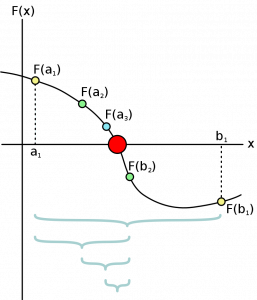# Bisection Method in C and C++

In this tutorial you will get program for bisection method in C and C++.

To find a root very accurately Bisection Method is used in Mathematics. Bisection method algorithm is very easy to program and it always converges which means it always finds root.

Bisection Method repeatedly bisects an interval and then selects a subinterval in which root lies. It is a very simple and robust method but slower than other methods.

It is also called Interval halving, binary search method and dichotomy method.

Bisection Method calculates the root by first calculating the mid point of the given interval end points.Image Source

### Bisection Method Procedure

The input for the method is a continuous function f, an interval [a, b], and the function values f(a) and f(b). The function values are of opposite sign (there is at least one zero crossing within the interval). Each iteration performs these steps:

1. Calculate the midpoint c = (a + b)/2

2. Calculate the function value at the midpoint, function(c).

3. If convergence is satisfactory (that is, a – c is sufficiently small, or f(c) is sufficiently small), return c and stop iterating.

4. Examine the sign of f(c) and replace either (a, f(a)) or (b, f(b)) with (c, f(c)) so that there is a zero crossing within the new interval.

### Pros and Cons

Advantage of the bisection method is that it is guaranteed to be converged and very easy to implement.

Disadvantage of bisection method is that it cannot detect multiple roots and is slower compared to other methods of calculating the roots.

## Program for Bisection Method in C

Output

a = -10.000000
b = 20.000000
Root = 5.000000
Root = -2.500000
Root = 1.250000
Root = -0.625000
Root = -1.562500
Root = -1.093750
Root = -0.859375
Root = -0.976563
Root = -1.035156
Root = -1.005859
Root = -0.991211
Root = -0.998535

Accurate Root calculated is = -0.998535

## Program for Bisection Method in C++

Comment below if you have any queries regarding above program for bisection method in C and C++.

### 9 thoughts on “Bisection Method in C and C++”

1.Surbhi Sorathiya

What is using namespace Std; ?

1.Md Al Amin Rahman

Using Using namespaces used to compile cout, cin, Endl

2.cos typing std:: every line is so annoying and hussle.

2.This program is be compiled in dev promgram so using namespace std; sould be define so say this program is c++

3.sir how can write a program using bisection method of function x-cos

4.how i can write a program using bisection method of function x-cosx

5.Bisection Method in C# :

using System;

namespace Application1
{
class Program
{
public double c;
public double func(double x)
{
return x * x * x – 2 * x * x + 3;
}
public void bisection(double a, double b, double e)
{
Program func = new Program();
if (func.func(a) * func.func(b) >= 0)
{
Console.WriteLine(“Incorrect a and b”);
return;
}
c = a;
while ((b – a) >= e)
{
c = (a + b) / 2;
if (func.func(c) == 0.0)
{
Console.WriteLine(“Root = ” + c);
break;
}
else if (func.func(c) * func.func(a) < 0)
{
Console.WriteLine("Root = " + c);
b = c;
}
else
{
Console.WriteLine("Root = " + c);
a = c;
}
}
}
public static void Main(string[] args)
{
double a, b, e;
Console.WriteLine("Enter the desired accuracy:");
Console.WriteLine("Enter the lower limit:");
Console.WriteLine("Enter the upper limit:");
1.6.